﻿ Energy Release Rate Calculated by J IntegralExamples | Product | Murata Software Co., Ltd.Example46Energy Release Rate Calculated by J Integral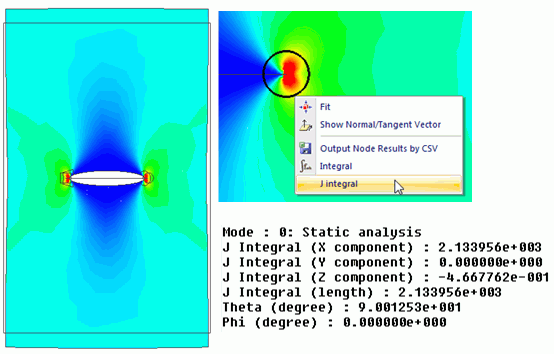General

• A plate with a crack is expanded and the mechanical stresses are analyzed.

• The energy release rate is calculated by J integral.

• Theoretically, the gapless crack has the infinite stress at its ends. Therefore, the stress is not a valid physical quantity to indicate the tendency of the crack growth. The energy release rate is a more common indicator for the purpose.
It is the rate at which the strain energy is released by the crack growth. The unit is [J/(m2)] (released strain energy / increased surface).

• Unless specified in the list below, the default conditions will be applied.

Analysis Space

 Item Settings Analysis Space 2D Model unit mm

Analysis Conditions

 Item Settings Solver Mechanical Stress Analysis [Galileo] Analysis Type Static analysis Options N/A

Model

The model is identical to the one on Exercise 34: Energy Release Rate.

The whole model is analyzed as J integral is performed for a path encircling the crack.

The crack is a wire body defined as imprinting body.

To make this exercise informative, the J integral paths for the left and right ends are set differently.

To show that the shapes don’t affect the simulation, they are shaped differently too.

< The left end >

Create a rectangle sheet body and imprint it on “Plate” body(*). The imprinted rectangle outline will be used as a J integral path for the left end. * See Segment Face for the details.

< The right end >

Create a circular sheet body, set the mesh size and define it as imprinting body.

See How to Set Body Attribute Partially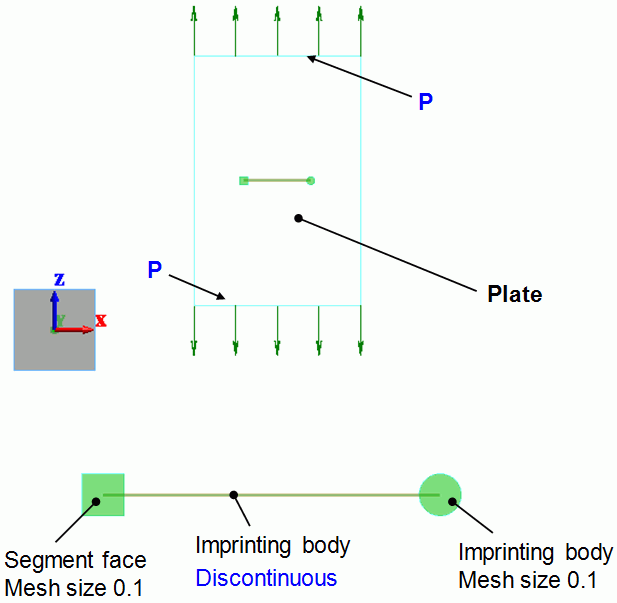Body Attributes and Materials

 Body Number/Type Body Attribute Name Material Name 0/Sheet Plate Fe

The thickness of sheet body is set to 1[m].

 Body Attribute Name Tab Settings Plate Thickness/Width Thickness of Sheet Body: 1000

Set Young’s modulus and Poisson’s ratio.

 Material Name Young’s modulus Poisson’s ratio. PlateMat* 70.56×109[Pa] 0.33

*Depends on the material.

Boundary Conditions

 Boundary Condition Name/Topology Tab Boundary Condition Type Settings Discontinuous/Edge Symmetry/Continuity Discontinuous N/A P/Edge Mechanical Pressure -98×10^6[Pa]

Results

The gradation contour diagram of maximum principal stresses around the right end is shown below.

The color scale range is 0 to 400MPa.

The calculated stresses are quite large. However they are neither accurate nor valid because they are supposed to be infinite theoretically as mentioned above and affected heavily by the shape of the mesh. (The stresses calculated at the locations more than several mesh sizes away from the end are valid.) To see the tendency of the crack growth, the energy release rate calculated by J integral should be examined.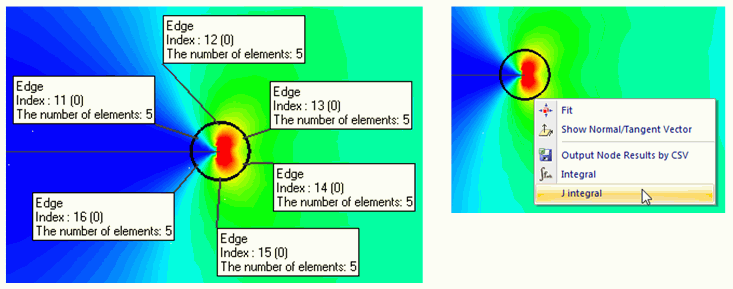Select the edges surrounding the right end (edge index: 11,12,13,14,15,16) and select “J integral” from the right-click menu.

The calculated J-integral vector will show up on the output window. The inner product of J-integral vector and the unit vector which indicates the crack growth direction is the energy release rate. Therefore the direction of J-integral vector is most likely that of the crack growth. The length of the J-integral vector is the energy release rate generated by the crack growth in the J-integral vector direction.

(Note) J integral is available only when the stress is set as field type.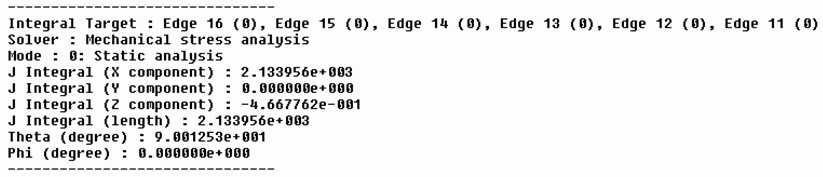The length is 2133.956 which is close to 2155.482 shown in the Exercise 34.

The direction indicated by θ = 90deg and φ = 0deg is shown on the following figure.

See [Components of Strain Energy Release Rate] in J Integral for details.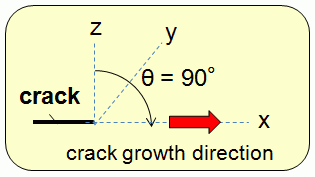The direction of J-integral vector is the positive X direction.

The gradation contour diagram of maximum principal stresses around the left end is shown below.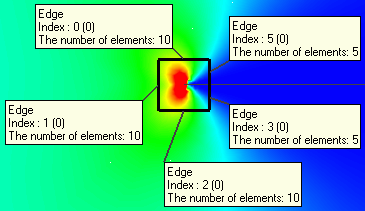Select the edges surrounding the left end (edge index: 0,1,2,3,5) and select “J integral” from the right-click menu.

The calculated J-integral vector will show up on the output window. The inner product of J-integral vector and the unit vector which indicates the crack growth direction is the energy release rate. Therefore the direction of J-integral vector is most likely that of the crack growth. The length of the J-integral vector is the energy release rate generated by the crack growth in the J-integral vector direction.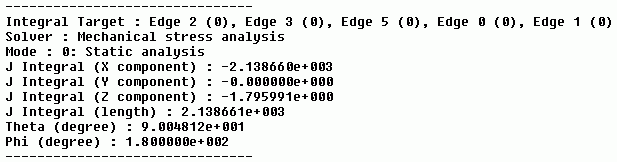The length of the vector is 2138.661. It is about the same as that of the right end calculated in the previous step (2133.956) despite the difference of the shape of J integral path.

The direction indicated by θ = 90deg and φ = 180deg is shown on following figure.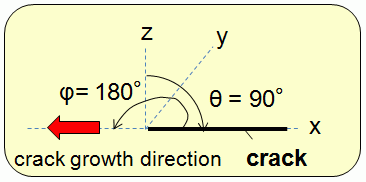The direction of J-integral vector is the negative X direction.

﻿